# Correlation in R: Pearson & Spearman with Matrix Example

A bivariate relationship describes a relationship -or correlation- between two variables, and . In this tutorial, we discuss the concept of correlation and show how it can be used to measure the relationship between any two variables.

There are two primary methods to compute the correlation between two variables.

• Pearson: Parametric correlation
• Spearman: Non-parametric correlation

## Pearson Correlation

The Pearson correlation method is usually used as a primary check for the relationship between two variables.

The coefficient of correlation , , is a measure of the strength of the linear relationship between two variables and . It is computed as follow: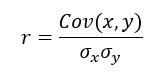Learn Java Programming with Beginners Tutorial

with

The correlation ranges between -1 and 1.

• A value of near or equal to 0 implies little or no linear relationship between and .
• In contrast, the closer comes to 1 or -1, the stronger the linear relationship.

We can compute the t-test as follow and check the distribution table with a degree of freedom equals to :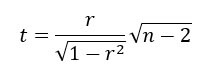## Spearman Rank Correlation

A rank correlation sorts the observations by rank and computes the level of similarity between the rank. A rank correlation has the advantage of being robust to outliers and is not linked to the distribution of the data. Note that, a rank correlation is suitable for the ordinal variable.

Spearman’s rank correlation, , is always between -1 and 1 with a value close to the extremity indicates strong relationship. It is computed as follow: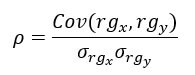with stated the covariances between rank and . The denominator calculates the standard deviations.

In R, we can use the cor() function. It takes three arguments, , and the method.

cor(x, y, method)

Arguments :

• x: First vector
• y: Second vector
• method: The formula used to compute the correlation. Three string values:
• “pearson”
• “kendall”
• “spearman”

An optional argument can be added if the vectors contain missing value: use = “complete.obs”

We will use the BudgetUK dataset. This dataset reports the budget allocation of British households between 1980 and 1982. There are 1519 observations with ten features, among them:

• wfood: share food share spend
• wfuel: share fuel spend
• wcloth: budget share for clothing spend
• walc: share alcohol spend
• wtrans: share transport spend
• wother: share of other goods spend
• totexp: total household spend in pound
• income total net household income
• age: age of household
• children: number of children

Example

library(dplyr) PATH <-“https://raw.githubusercontent.com/guru99-edu/R-Programming/master/british_household.csv” data <-read.csv(PATH) filter(income < 500) mutate(log_income = log(income), log_totexp = log(totexp), children_fac = factor(children, order = TRUE, labels = c(“No”, “Yes”))) select(-c(X,X.1, children, totexp, income)) glimpse(data)

Code Explanation

• We first import the data and have a look with the glimpse() function from the dplyr library.
• Three points are above 500K, so we decided to exclude them.
• It is a common practice to convert a monetary variable in log. It helps to reduce the impact of outliers and decreases the skewness in the dataset.

Output:

## Observations: 1,516## Variables: 10 ## \$ wfood 0.4272, 0.3739, 0.1941, 0.4438, 0.3331, 0.3752, 0… ## \$ wfuel 0.1342, 0.1686, 0.4056, 0.1258, 0.0824, 0.0481, 0… ## \$ wcloth 0.0000, 0.0091, 0.0012, 0.0539, 0.0399, 0.1170, 0… ## \$ walc 0.0106, 0.0825, 0.0513, 0.0397, 0.1571, 0.0210, 0… ## \$ wtrans 0.1458, 0.1215, 0.2063, 0.0652, 0.2403, 0.0955, 0… ## \$ wother 0.2822, 0.2444, 0.1415, 0.2716, 0.1473, 0.3431, 0… ## \$ age 25, 39, 47, 33, 31, 24, 46, 25, 30, 41, 48, 24, 2… ## \$ log_income 4.867534, 5.010635, 5.438079, 4.605170, 4.605170,… ## \$ log_totexp 3.912023, 4.499810, 5.192957, 4.382027, 4.499810,… ## \$ children_fac Yes, Yes, Yes, Yes, No, No, No, No, No, No, Yes, …

We can compute the correlation coefficient between income and wfood variables with the “pearson” and “spearman” methods.

cor(data\$log_income, data\$wfood, method = “pearson”)

output:

##  -0.2466986

cor(data\$log_income, data\$wfood, method = “spearman”)

Output:

## Correlation Matrix

The bivariate correlation is a good start, but we can get a broader picture with multivariate analysis. A correlation with many variables is pictured inside a correlation matrix . A correlation matrix is a matrix that represents the pair correlation of all the variables.

The cor() function returns a correlation matrix. The only difference with the bivariate correlation is we don’t need to specify which variables. By default, R computes the correlation between all the variables.

Note that, a correlation cannot be computed for factor variable. We need to make sure we drop categorical feature before we pass the data frame inside cor().

A correlation matrix is symmetrical which means the values above the diagonal have the same values as the one below. It is more visual to show half of the matrix.

We exclude children_fac because it is a factor level variable. cor does not perform correlation on a categorical variable.

# the last column of data is a factor level. We don’t include it in the code mat_1 <-as.dist(round(cor(data[,1:9]),2)) mat_1

Code Explanation

• cor(data): Display the correlation matrix
• round(data, 2): Round the correlation matrix with two decimals
• as.dist(): Shows the second half only

Output:

## wfood wfuel wcloth walc wtrans wother age log_income ## wfuel 0.11 ## wcloth -0.33 -0.25 ## walc -0.12 -0.13 -0.09 ## wtrans -0.34 -0.16 -0.19 -0.22 ## wother -0.35 -0.14 -0.22 -0.12 -0.29 ## age 0.02 -0.05 0.04 -0.14 0.03 0.02 ## log_income -0.25 -0.12 0.10 0.04 0.06 0.13 0.23 ## log_totexp -0.50 -0.36 0.34 0.12 0.15 0.15 0.21 0.49

### Significance level

The significance level is useful in some situations when we use the pearson or spearman method. The function rcorr() from the library Hmisc computes for us the p-value. We can download the library from conda and copy the code to paste it in the terminal:

conda install -c r r-hmisc

The rcorr() requires a data frame to be stored as a matrix. We can convert our data into a matrix before to compute the correlation matrix with the p-value.

library(“Hmisc”) data_rcorr <-as.matrix(data[, 1: 9]) mat_2 <-rcorr(data_rcorr) # mat_2 <-rcorr(as.matrix(data)) returns the same output

The list object mat_2 contains three elements:

• r: Output of the correlation matrix
• n: Number of observation
• P: p-value

We are interested in the third element, the p-value. It is common to show the correlation matrix with the p-value instead of the coefficient of correlation.

p_value <-round(mat_2[[“P”]], 3) p_value

Code Explanation

• mat_2[[“P”]]: The p-values are stored in the element called P
• round(mat_2[[“P”]], 3): Round the elements with three digits

Output:

wfood wfuel wcloth walc wtrans wother age log_income log_totexp wfood NA 0.000 0.000 0.000 0.000 0.000 0.365 0.000 0 wfuel 0.000 NA 0.000 0.000 0.000 0.000 0.076 0.000 0 wcloth 0.000 0.000 NA 0.001 0.000 0.000 0.160 0.000 0 walc 0.000 0.000 0.001 NA 0.000 0.000 0.000 0.105 0 wtrans 0.000 0.000 0.000 0.000 NA 0.000 0.259 0.020 0 wother 0.000 0.000 0.000 0.000 0.000 NA 0.355 0.000 0 age 0.365 0.076 0.160 0.000 0.259 0.355 NA 0.000 0 log_income 0.000 0.000 0.000 0.105 0.020 0.000 0.000 NA 0 log_totexp 0.000 0.000 0.000 0.000 0.000 0.000 0.000 0.000 NA

## Visualize Correlation Matrix

A heat map is another way to show a correlation matrix. The GGally library is an extension of ggplot2. Currently, it is not available in the conda library. We can install directly in the console.

install.packages(“GGally”)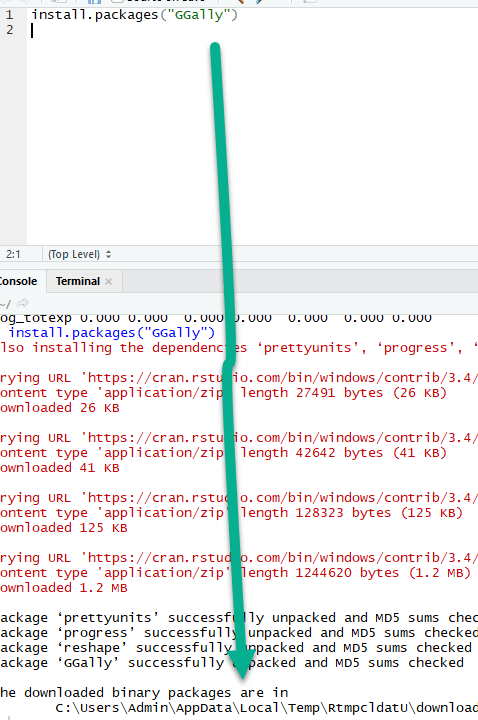The library includes different functions to show the summary statistics such as the correlation and distribution of all the variables in a matrix.

The ggcorr() function has lots of arguments. We will introduce only the arguments we will use in the tutorial:

The function ggcorr

ggcorr(df, method = c(“pairwise”, “pearson”), nbreaks = NULL, digits = 2, low = “#3B9AB2”, mid = “#EEEEEE”, high = “#F21A00”, geom = “tile”, label = FALSE, label_alpha = FALSE)

Arguments:

• df : Dataset used
• method : Formula to compute the correlation. By default, pairwise and Pearson are computed
• nbreaks : Return a categorical range for the coloration of the coefficients. By default, no break and the color gradient is continuous
• digits : Round the correlation coefficient. By default, set to 2
• low : Control the lower level of the coloration
• mid : Control the middle level of the coloration
• high : Control the high level of the coloration
• geom : Control the shape of the geometric argument. By default, “tile”
• label : Boolean value. Display or not the label. By default, set to `FALSE`

### Basic heat map

The most basic plot of the package is a heat map. The legend of the graph shows a gradient color from - 1 to 1, with hot color indicating strong positive correlation and cold color, a negative correlation.

library(GGally) ggcorr(data)

Code Explanation

• ggcorr(data): Only one argument is needed, which is the data frame name. Factor level variables are not included in the plot.

Output: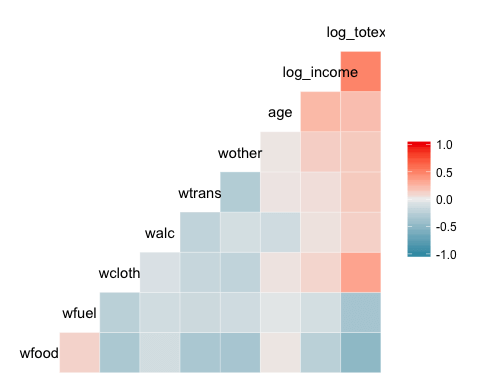### Add control to the heat map

We can add more controls to the graph.

ggcorr(data, nbreaks = 6, low = “steelblue”, mid = “white”, high = “darkred”, geom = “circle”)

Code Explanation

• nbreaks=6: break the legend with 6 ranks.
• low = “steelblue”: Use lighter colors for negative correlation
• mid = “white”: Use white colors for middle ranges correlation
• high = “darkred”: Use dark colors for positive correlation
• geom = “circle”: Use circle as the shape of the windows in the heat map. The size of the circle is proportional to the absolute value of the correlation.

Output: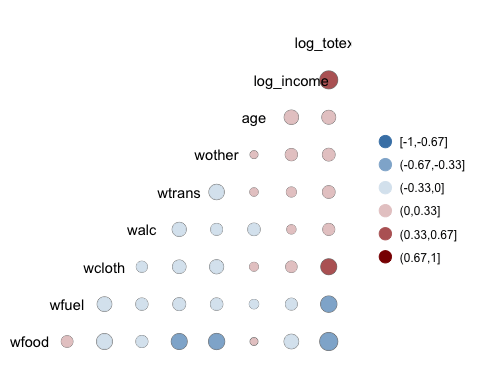### Add label to the heat map

GGally allows us to add a label inside the windows.

ggcorr(data, nbreaks = 6, label = TRUE, label_size = 3, color = “grey50”)

Code Explanation

• label = TRUE: Add the values of the coefficients of correlation inside the heat map.
• color = “grey50”: Choose the color, i.e. grey
• label_size = 3: Set the size of the label equals to 3

Output: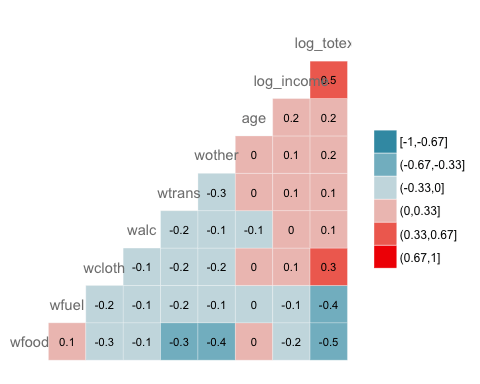### ggpairs

Finally, we introduce another function from the GGaly library. Ggpair. It produces a graph in a matrix format. We can display three kinds of computation within one graph. The matrix is a dimension, with equals the number of observations. The upper/lower part displays windows and in the diagonal. We can control what information we want to show in each part of the matrix. The formula for ggpair is:

ggpair(df, columns = 1: ncol(df), title = NULL, upper = list(continuous = “cor”), lower = list(continuous = “smooth”), mapping = NULL)

Arguments :

• df : Dataset used
• columns : Select the columns to draw the plot
• title : Include a title
• upper : Control the boxes above the diagonal of the plot. Need to supply the type of computations or graph to return. If continuous = “cor”, we ask R to compute the correlation. Note that, the argument needs to be a list. Other arguments can be used, see the vignette for more information.
• Lower : Control the boxes below the diagonal.
• Mapping : Indicates the aesthetic of the graph. For instance, we can compute the graph for different groups.

### Bivariate analysis with ggpair with grouping

The next graph plots three information:

• The correlation matrix between log_totexp, log_income, age and wtrans variable grouped by whether the household has a kid or not.
• Plot the distribution of each variable by group
• Display the scatter plot with the trend by group

library(ggplot2) ggpairs(data, columns = c(“log_totexp”, “log_income”, “age”, “wtrans”), title = “Bivariate analysis of revenue expenditure by the British household”, upper = list(continuous = wrap(“cor”, size = 3)), lower = list(continuous = wrap(“smooth”, alpha = 0.3, size = 0.1)), mapping = aes(color = children_fac))

Code Explanation

• columns = c(“log_totexp”, “log_income”, “age”, “wtrans”): Choose the variables to show in the graph
• title = “Bivariate analysis of revenue expenditure by the British household”: Add a title
• upper = list(): Control the upper part of the graph. I.e. Above the diagonal
• continuous = wrap(“cor”, size = 3)): Compute the coefficient of correlation. We wrap the argument continuous inside the wrap() function to control for the aesthetic of the graph ( i.e. size = 3) -lower = list(): Control the lower part of the graph. I.e. Below the diagonal.
• continuous = wrap(“smooth”,alpha = 0.3,size=0.1): Add a scatter plot with a linear trend. We wrap the argument continuous inside the wrap() function to control for the aesthetic of the graph ( i.e. size=0.1, alpha=0.3)
• mapping = aes(color = children_fac): We want each part of the graph to be stacked by the variable children_fac, which is a categorical variable taking the value of 1 if the household does not have kids and 2 otherwise

Output: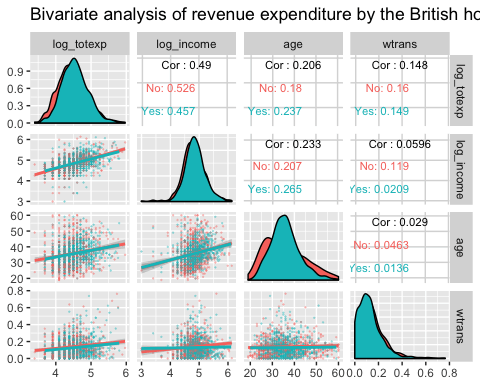### Bivariate analysis with ggpair with partial grouping

The graph below is a little bit different. We change the position of the mapping inside the upper argument.

ggpairs(data, columns = c(“log_totexp”, “log_income”, “age”, “wtrans”), title = “Bivariate analysis of revenue expenditure by the British household”, upper = list(continuous = wrap(“cor”, size = 3), mapping = aes(color = children_fac)), lower = list( continuous = wrap(“smooth”, alpha = 0.3, size = 0.1)) )

Code Explanation

• Exact same code as previous example except for:
• mapping = aes(color = children_fac): Move the list in upper = list(). We only want the computation stacked by group in the upper part of the graph.

Output: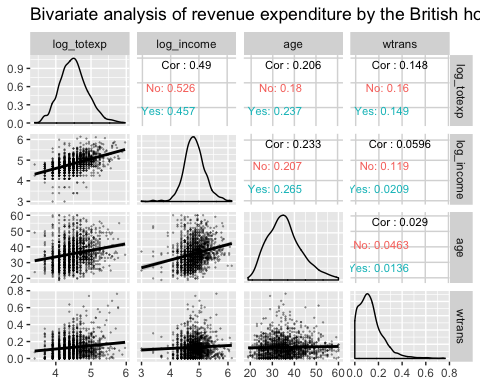## Summary

We can summarize the function in the table below:

library Objective method code
Base bivariate correlation Pearson cor(dfx2, method = “pearson”)
Base bivariate correlation Spearman cor(dfx2, method = “spearman”)
Base Multivariate correlation pearson cor(df, method = “pearson”)
Base Multivariate correlation Spearman cor(df, method = “spearman”)
Hmisc P value rcorr(as.matrix(data[,1:9]))[[“P”]]
Ggally heat map ggcorr(df)
Multivariate plots cf code below